## Ramanujan g- and G-Functions

Following Ramanujan(1913-14), write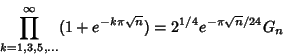(1)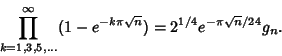(2)

These satisfy the equalities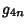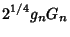(3)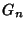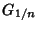(4)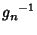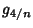(5)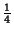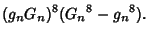(6)and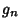can be derived using the theory of Modular Functions and can always be expressed as roots of algebraic equations whenis Rational. For simplicity, Ramanujan tabulatedforEven andforOdd. However, (6) allowsandto be solved for in terms ofand, giving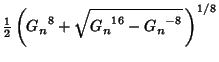(7)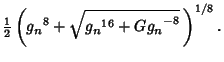(8)

Using (3) and the above two equations allowsto be computed in terms ofor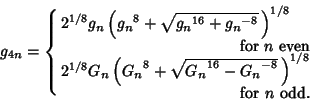(9)

In terms of the Parameter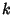and complementary Parameter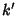,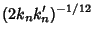(10)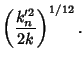(11)

Here,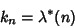(12)

is the Elliptic Lambda Function, which gives the value offor which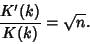(13)

Solving for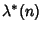gives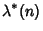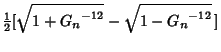(14)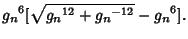(15)

Analytic values for small values ofcan be found in Ramanujan(1913-1914) and Borwein and Borwein (1987), and have been compiled in Weisstein (1996). Ramanujan(1913-1914) contains a typographical error labeling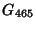as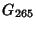.

References

Borwein, J. M. and Borwein, P. B. Pi & the AGM: A Study in Analytic Number Theory and Computational Complexity. New York: Wiley, pp. 139 and 298, 1987.

Ramanujan, S. Modular Equations and Approximations to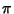.'' Quart. J. Pure. Appl. Math. 45, 350-372, 1913-1914.Weisstein, E. W. Elliptic Singular Values.'' Mathematica notebook EllipticSingular.m.